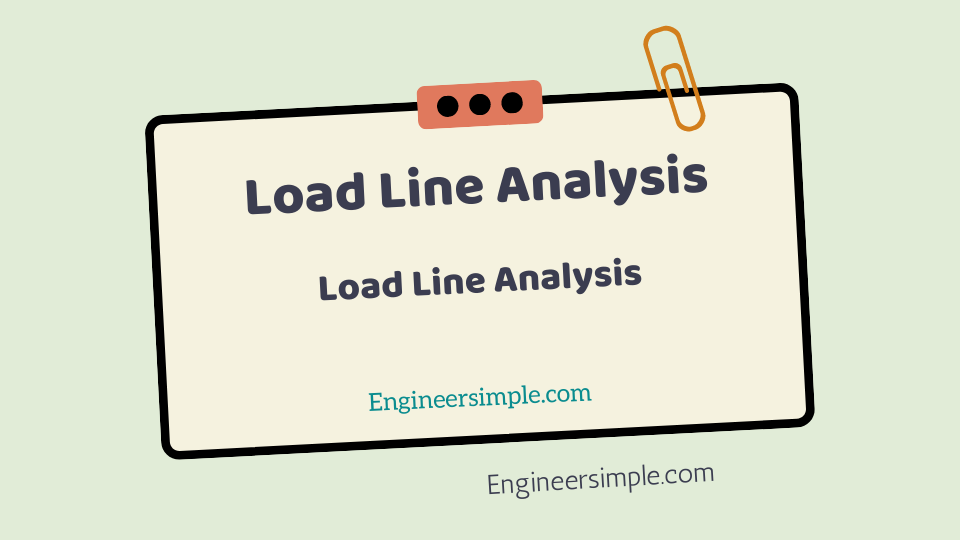Load Line Analysis - Engineer Simple

#The output characteristics are determined experimentally and indicate the relation between VCE and IC. However, the same information can be obtained in a much simpler way by representing the mathematical relation between IC and VCE graphically.

## The relationship between VCE and IC

As discussed before,the relationship between VCE and IC is linear so that it can be represented by a straight line on the output characteristics. This is know as a load line. The points lying on the load line give the possible values of VCE and IC in the
output circuit. As in a transistor circuit both d.c. and a.c. conditions exist, therefore, there are two
types of load lines, namely ; d.c. load line and a.c. load line. The former determines the locus of IC
and VCE in the zero signal conditions and the latter shows these values when the signal is apply.

It is the line on the output characteristics of a transistor circuit which gives
the values of IC and VCE corresponding to zero signal or d.c. conditions

Consider the transistor Load Line Analysis amplifier shown in Fig. 1. In the absence of signal, d.c. conditions
prevail in the circuit as shown in Fig. 2(i). Referring to this circuit and applying Kirchhoff’s
voltage law,

##### VCE = VCC − IC RC − IE REor VCE = VCC − IC (RC + RE)

As for a given circuit, VCC and (RC + RE) are constant, therefore, it is a first degree *equation and
can be represented by a straight line on the output characteristics. This is know as d.c. load line and
determines the loci of VCE and IC points in the zero signal conditions. The d.c. load line can be readily
plotted by locating two end points of the straight line.

The value of VCE will be maximum when IC = 0. Therefore, by putting IC = 0 in exp. (i), we get,
Max. VCE = VCC
This locates the first point B (OB = VCC) of the d.c. load line.

This equation is know as load line equation since it relates the collector-emitter voltage (VCE) to the
collector current (IC) flowing through the load.

The value of IC will be maximum when VCE = 0.
∴ Max. IC = CC
C E
V
R R +
This locates the second point A (OA = VCC/RC + RE) of the d.c. load line. By joining points A and
B, d.c. load line AB is constructed [See Fig. 2 (ii)].